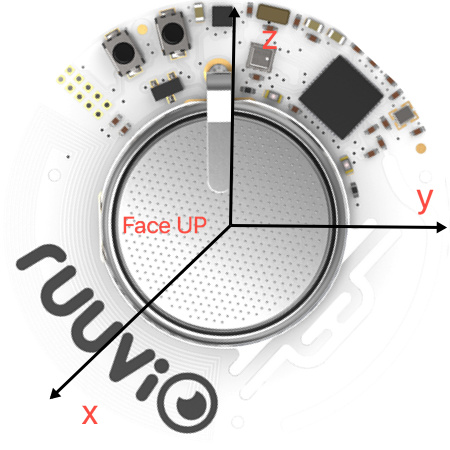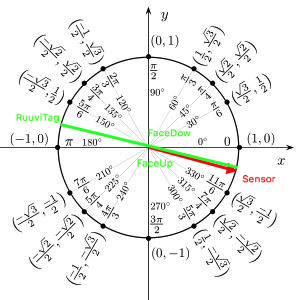# Accelerometer: get a value as angle (pitch and roll)

Hi there!

I need to have the information of the the angle of inclination of the RuuviTag+ in degree (0-360).
In order to make that, I’m trying to use a past code made for ADXL335 accelerometer. It has coded to get the pitch by using Z and X values and some transformation based on Vreference and so on… I don’t have a clear idea on how it works. The code that I made until now is that:

``````setInterval(function() {
on = !on;
//console.log(Ruuvitag.getAccelData().x);
accValues = Ruuvitag.getAccelData();
var x_g_value = ((((accValues.x * 5)/1024) - 1.65 ) / 0.330 );
var y_g_value = ( ( ((accValues.y * 5)/1024) - 1.65 ) / 0.330 );
var z_g_value = ((((accValues.z * 5)/1024) - 1.80 ) / 0.330 );

var pitch = ( ( (Math.atan2(z_g_value,x_g_value) * 180) / 3.14 ) + 180 );
//console.log(pitch);
//LED1.write(on);
}, 500);
``````

Obviously however the values are not good for Ruuvitag+. Someone could help me?

Hi,

Check `public static RuuviMeasurement calculateAllValues`

Hi Otso,

I tried, but it doesn’t work. Here my code:

``````var Ruuvitag = require("Ruuvitag");
Ruuvitag.setAccelOn(true);

function totalAcceleration(accelerationX,accelerationY,accelerationZ) {
if (accelerationX === null || accelerationY === null || accelerationZ === null) {
return null;
}
return Math.sqrt(accelerationX * accelerationX + accelerationY * accelerationY + accelerationZ * accelerationZ);
}

function angleBetweenVectorComponentAndAxis(vectorComponent, vectorLength) {
if (vectorComponent === null || vectorLength === null || vectorLength === 0) {
return null;
}
var angle = Math.acos(vectorComponent / vectorLength);
//return Math.toDegrees(angle);
}

var pi = Math.PI;
}
setInterval(function() {
accValues = Ruuvitag.getAccelData();
accelerationX = accValues.x;
accelerationY = accValues.y;
accelerationZ = accValues.z;
accelerationTotal = totalAcceleration(accelerationX,accelerationY,accelerationZ);
accelerationAngleFromX = angleBetweenVectorComponentAndAxis(accelerationX,accelerationTotal);
accelerationAngleFromY = angleBetweenVectorComponentAndAxis(accelerationY,accelerationTotal);
accelerationAngleFromZ = angleBetweenVectorComponentAndAxis(accelerationZ,accelerationTotal);

console.log("X: " + accelerationAngleFromX + "\tY: " + accelerationAngleFromY + "\tZ: " + accelerationAngleFromZ);
}, 500);
``````

Theoretically, it should show an angle between 0 - 360 and instead also shows negative values and strange behavior.
Thanks.

Hello,

The `accelerationAngleFromX` should correspond to your `x_g_value`, assuming that the RuuviTag is stationary and only acceleration is the gravity. You can then use `var pitch = ( ( (Math.atan2(z_g_value,x_g_value) * 180) / 3.14 ) + 180 );` (or similar, I’ve not checked what the result would be).

Hello otso,

The angle actually shown is a value between 0 and 360, but it is not the true value. It has a strange behavior.
Is that strange behaviour maybe due to an not full compatibility with Espruino on this specific case?
What means the value that we get from accValues? Like accValues.x?
For me it is really important to take the value of the angle instant by instant with a certain reliability, as it will be the basis of my project.
Thanks for help!

Hello,

Could you be more specific?
Where your RuuviTag is installed, what kind of values you’re expecting and what you’re getting?

The code is:

``````var Ruuvitag = require("Ruuvitag");
Ruuvitag.setAccelOn(true);

function totalAcceleration(accelerationX,accelerationY,accelerationZ) {
if (accelerationX === null || accelerationY === null || accelerationZ === null) {
return null;
}
return Math.sqrt(accelerationX * accelerationX + accelerationY * accelerationY + accelerationZ * accelerationZ);
}

function angleBetweenVectorComponentAndAxis(vectorComponent, vectorLength) {
if (vectorComponent === null || vectorLength === null || vectorLength === 0) {
return null;
}
var angle = Math.acos(vectorComponent / vectorLength);
//return Math.toDegrees(angle);
}

var pi = Math.PI;
}
setInterval(function() {
accValues = Ruuvitag.getAccelData();
accelerationX = accValues.x;
accelerationY = accValues.y;
accelerationZ = accValues.z;
accelerationTotal = totalAcceleration(accelerationX,accelerationY,accelerationZ);
accelerationAngleFromX = angleBetweenVectorComponentAndAxis(accelerationX,accelerationTotal);
accelerationAngleFromY = angleBetweenVectorComponentAndAxis(accelerationY,accelerationTotal);
accelerationAngleFromZ = angleBetweenVectorComponentAndAxis(accelerationZ,accelerationTotal);
pitch = (((Math.atan2(accelerationAngleFromZ,accelerationAngleFromX) * 180) / 3.14 ) + 180 );
console.log("Pitch: " + pitch);
}, 500);
``````

Here are the images of the axes with respect to the sensor:At this point I made my measurements and I got (angle in degree):
Standing on the table, FaceUp to the ceiling: 180 - 182 / 358-359
FaceUp to me: 92 - 93 / 263 - 264
FaceUp down: 2 - 5 / 173 -174
FaceUp outwards: 271 / 88

Moreover, having the FaceUp towards the ceiling and tilting it towards me, the angle should be 135° in reality the one measured is 225. In fact, the values that should be between 180 and 90 but those measured are 180 and 270.

This should be because of how the sensor feels the gravity. What I need is a unique angle value following the counterclockwise fencing (see this picture).I hope the problem is now clearer. Thank you

Just to clarify:

Do you get 180 degree “jumps” on the measurement while sensor is still?

This sounds like you’d have 90 degree offset in the results. The Z-axis positive direction is to “FaceUp”.

No, the value is fixed. From 182 pass to 358 only if I tilt it a few degrees manually and then fix it in that position.

Hello,

Maybe this STMicro article about 3-axis sensing, especially equations 6. and 7. would help?

Or maybe you could drop the `angleBetweenVectorComponentAndAxis` as it seems that the original code meant “Acceleration of axis x in g”, which is actually the same raw value which RuuviTag gives

Hello,

My problem is not solved.

``````accValues = Ruuvitag.getAccelData();
console.log("X: " + accValues.x + "\tY: " + accValues.y + "\tZ: " + accValues.z);
``````

Let’s start again from the beginning: what do these data mean? What is the unit of measurement?
I don’t understand what these values are. Could you please explain?

My problem is to get, in some way, instant by instant the angle of inclination measured by the RuuviTag, measured in degrees between 0 and 360, so I can perform some operations such as counting the laps of the device.
Thanks for help.

Hello,

The raw values are in milli-g, thousandths of a g.

I’m not too familiar with the mathematics on how to convert the acceleration to roll and yaw, essentially all I can do there is to read through application notes and check if they have an algorithm that would work

Hello,

I noticed that the RuuviTag takes values from the accelerometer every 900 - 950 ms. In fact, the value does not change within that interval of time. Why this limit? Is it possible to change it to increase the refresh rate through Espruino?
I hope at least it can be modified via firmware (I bought the devkit that has to arrive).

Thanks.

I’m not sure how Espruino configures the sample rate, but I’m sure it’s configurable as the Espruino sets it to 1 Hz. LIS2DH12 supports at least 1, 10, 25, 50, 100, 200 and 400 if I recall correctly.